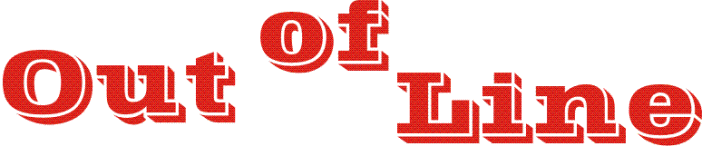By Ronnie Brown, Professor Emeritus, University of Wales Bangor.

## Contents. (The paper is split into 3 web pages for browsing)

 First page 1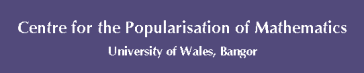This web version of the paper includes images from the popularisation web site (click on the image) and accompanying CD. Contact the author for more information and to obtain a copy of the CD. For mathematicians: "From groups to groupoids: a brief survey" (ref ) ; BOOK: Topology and Groupoids. R. Brown 2006. 3rd edition.Order online. 2 4 This page IV. Space. 7 V. Motion.      Pivoted Lines and the Möbius Band.      The Projective Plane and the Brehm Model.      The Dirac String Trick.      The Double Pendulum.      Paths and Knot Spaces. 9 Last page. 15 18 22 IX.Implications. References. 24 PDF.PDF version 24pp, 700KB OPEN/DOWNLOAD.

# IV. Space

In the time of Euclid, geometry was seen as the study of our three dimensional space, and the properties of this space were reduced to some "self evident" postulates and axioms, from which further properties (constructions, propositions and theorems) were deduced.

The mathematical view of "space" has changed radically since then. Now mathematics abounds in structures called a "space" of some kind. The dimensions of these mathematical spaces range from zero to infinity, even to what are called uncountable infinities, and the notion of dimension has been enlarged to include fractal spaces, with fractional dimensions, which are used in the study of chaos. The applications of mathematical space range all over science, from fundamental particle theory, to statistics. A relation with the artist's view of space is shown in the works of the sculptor/mathematician Helaman Ferguson , and the sculptor John Robinson .

It is necessary to give some impression of the intentions of a mathematical space. I will illustrate this first in relation to the notion of symmetry, by borrowing some results of Stuart Robertson, of Southampton University, who has supplied the design of the models for 22 objects displayed in this Address, which we call Robertson's cuboids. All of these 22 objects have 8 vertices and 12 edges, and their 6 faces are plane quadrilaterals. Some of these cuboids are more symmetrical than others. In order to explain this, it is convenient to move down a dimension and look at squaroids (again, Robertson's term), that is the plane quadrilaterals. Robertson classifies these into symmetry types .

TABLE 1
OBJECT

NAME

SYMMETRY

DEFICIENCY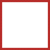Square

8

0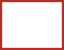Rectangle

4

1Rhombus

4

1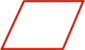Parallelogram

2

2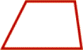Isosceles trapezium

2

2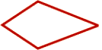Deltoid

2

2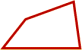1

4

The column "symmetry" gives the number of symmetry operations, including the identity. The column "deficiency" is more important for our purposes.

First, two objects are called "similar" (in the  familiar "Euclidean" sense of having the same geometry) if one can be transformed to the other by reflection, rotation, translation or uniform stretching or contracting in all directions. So all squares are similar, as are all cubes.

Now we have to imagine a "space" of all these symmetry types. In this space, there is only one point for the square, since all squares are similar. So the "deficiency" of the square is the dimension of a point, which is 0. However two rectangles are similar if and only if they have the same ratio of the longest to the shortest side. This ratio, a number greater than or equal to 1, specifies the similarity class of the rectangle completely. The "space" of these types of rectangles can be pictured as a part of a line starting at 1, which represents the square, and going away indefinitely......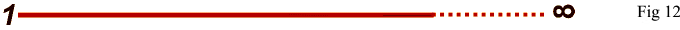Since a line has dimension 1, or "one degree of freedom", the rectangle is said to have deficiency 1. A rhombus is specified up to similarity by an angle between 0° and 90° . So the space of types of rhombuses can be pictured as a part of a line:where 0° represents a line segment, and 90° represents a square. The type of a parallelogram is specified by the ratio of the longest to the shortest side and by an angle between 0° and 90° . The parallellogram thus has deficiency 2 and its symmetry space looks as in Fig 14:where the bottom line is omitted since for angle 0° a parallelogram becomes a line segment, of deficiency 0. We leave the reader to picture the symmetry spaces of the other squaroids. The spaces of all these squaroids fit together into one big space of which Fig 13 (rhombuses, rectangles, parallellograms, and squares at the corner) is a part.

Now let us move to three dimensions. The cube has already been pictured. It is one of the five regular Platonic solids whose explanation was one of the aims of Euclid's Elements of Geometry. All the Platonic solids have deficiency zero: for example, any two dodecahedra are similar to each other. By contrast, two rectangular blocks are similar if they have the same ratios of their edges, in order of increasing length. So the symmetry type of the rectangular block is specified by two numbers greater than or equal to 1. Thus the symmetry space of the rectangular block has dimension 2, and the rectangular block has deficiency 2. There is a cuboid with no symmetry at all, and there is a cuboid with only three symmetries. Each of these cuboids has an associated "symmetry space", consisting of all those cuboids of the same symmetry type. The dimensions of these spaces varies. For some of these spaces, not much is known apart from their dimension. The possible dimensions are from 0 to 11, excluding 8, 9, 10. The 22 objects represent all the possible types of symmetry of cuboids.

For us, these cuboids illustrate at once the two concepts of symmetry and of mathematical space, and also illustrate the use in mathematics of the notion of classification.

# V. Motion

We have illustrated the idea that the points of a "mathematical space" can represent data. Change of data may be represented by a motion in that space. This is a profound idea that has had a widespread influence on mathematics and mathematical applications over the 20th century.

The next illustration of the notion of mathematical space presented during the lecture was a silent video created using computer graphics at IBMUK Scientific Research, Winchester, (programmed by Ramen Sen). Called 'Pivoted Lines and the Möbius Band`, here is an account of the video (figs 15-26). The video itself (1.47MB) is given below; the commentary should be given as follows.

#### Pivoted lines and the Möbius Band .Fig 15. We start with a moving pivoted line in our 3-dimensional space, moving either randomly or in a regular fashion. It is important for our purposes that this line has no direction, so that rotation through 180º brings it back to itself.We start to simplify our picture. We introduce a sphere, centred on our pivot. We need only part of the line to specify all of it, and so we omit the part of the line outside the sphere. The line meets the sphere in two points, which we show as discs.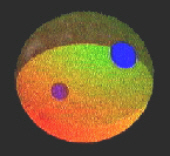These two moving discs contain all the information about the position of the line, and so we can omit the line, leaving the two discs. The two moving discs still record more information than is necessary. We can recover the line from the information contained in half the sphere (since we know the centre of the sphere). So we gradually eat away half of the sphere, leaving a hemisphere and one moving disc.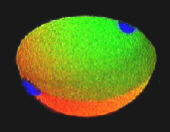As this disc moves across the equator, it appears on the opposite side of the hemisphere. So we represent the moving pivoted line in our usual space by the picture of a point or disc moving on a hemisphere, with the rule that opposite points of the equatorial circle of the hemisphere are to be thought of as glued or sewn together, since the disc jumps from one point of the equator to the opposite. This gluing cannot be done in three dimensions, so instead what we do is just record the information that this gluing is to be done, as shown by the motion of the disc.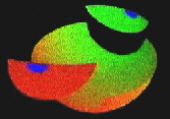This hemisphere with parts glued together is a complicated space. There is another useful picture of it, which brings in the Möbius Band. (right). Take small semicircular pieces, including parts of the equator, out of opposite sides of the hemisphere.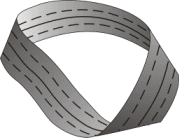Fig 27. Möbius Band.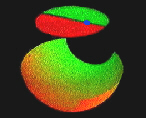We glue these pieces together, with a twist, and they form a disc.We gradually deform the remainder so that it becomes more like a strip.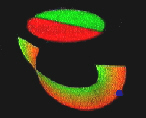Now we can glue together those parts of this strip which should have have been glued as part of the hemisphere. So there has to be a twist as we glue, and we get the famous Band. This extraordinary surface has only one side and one edge, and the edge is like a circle. We will say more about the Möbius Band later. The blue dot is our disk.We have replaced our original picture of a moving pivoted line in 3-dimensional space by a point (or disc) moving on a space which consists of a Möbius Band and a disc. The edges of the disc and the Möbius Band are circles, and these circular edges are to be thought of as glued together. Again, we cannot do this gluing or sewing in our real three dimensional space, but we illustrate it by showing our moving disc hopping instantaneously from the edge of the Möbius Band to the disc, and back again (a traced track is shown as the blue line).This picture of our mathematical space is convenient for representing types of motion of our pivoted line. The disc now leaves a trail, which joins up to form what we call a loop. This loop represents a regular and repetitive motion of the original line. Now we can deform the loop to show different motions.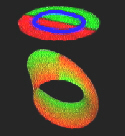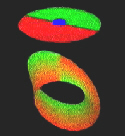Fig 26. The loop expands off the Möbius Band, onto the disc, and finally down to a point. This point corresponds to a stationary line.

The movement of this loop shows how our two parts of the space are joined. It also shows us the advantages of the new picture: the changes of types of motion are more easily represented in our new model than in our original picture. Our new model allows for a global picture of particular motions of the line in 3-dimensional space.

Another important aspect is that the movements of this loop not only describe different motions, but also give us information on the mathematical space which represents the positions of the line. We are interested not only in movements, but in movements of movements, and so on, and this is an intimation of the higher dimensional theory.

Here is the silent movie which is the origin of the above account:

#### The 'Projective Plane' and the Brehm Model.

There is more to be said about the space represented in the video. Mathematicians call it the "projective plane". This term is used because of its relations to the notion of projection in technical drawing, which concerns looking at a model or object from different viewpoints. There is no way of building the projective plane in 3-dimensional space. This is a mathematical fact, a theorem, but a little experiment will convince you that a disc of cloth cannot be sewn onto the edge of a Möbius Band. The way in which an attempt at this process gets tangled up shows that there might be a model with self intersections. The first of these was produced by Boy, a student of Hilbert, at the end of the last century.Fig 28. A remarkable model with flat faces has recently been discovered by U Brehm . First, one makes three horses heads (Fig 28). The crucial feature of these is that the lengths of the parts AB and CD are to be the same. These three are glued together so that the part AB of one is attached to CD of another (Fig 29). The result is a Möbius Band.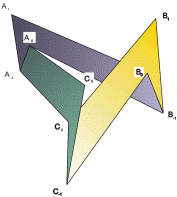Fig 29.

Now to form the projective plane, seven more triangles have to be added. Four of them are added on the outside and cause no problems, but three have to be added to intersect each other inside, like three planes meeting at a point. Instructions for making this model are in Brehm's article.
 The model of the Möbius Band was the inspiration for a sculpture Journeys (right) by John Robinson . One should not forget the people involved in science, and there is a mystery about Boy. The last that is known of him is a 1902 letter in the Göttingen archives, written to Hilbert when Boy was 23, saying he was looking forward to discussions. Thereafter, he disappears from sight, and there is no record of his death even in the archives of his home town. (There is a rumour he went to the USA.)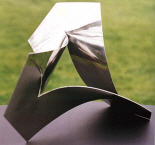Fig 30.

#### Dirac's string trick

At one point in the sequence on the video, we have a loop which goes twice round the Möbius Band, and then this loop is deformed off the band and over to the disc, and then to a small loop, and finally to a point, which represents a stationary line. This is related to the famous Dirac String Trick.

Make two squares out of board and draw a large arrow on one of them. Attach the four corners of one square to the four corners of the other by string or, even better, differently coloured ribbons, as shown in Fig 19. It is good to use bulldog clips for the attachment in case the apparatus gets tangled in the next part!
 Now rotate the top square by 360º about a vertical axis. The ribbons will become somewhat tangled, and it is not possible to untangle them without unrotating the square. There is an old adage that there is nothing so complicated that it cannot, with sufficient trouble, be made more complicated. So we rotate through another 360º, a total of 720º. Contrary to the adage, it is now possible to untangle the ribbons, without further rotation of the square! Another version of the Dirac string trick has been called the Philippine wine glass trick. A glass of water held in the hand can be rotated continuously through 720º without spilling any water.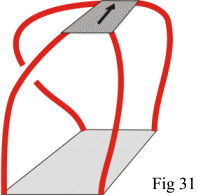These geometrical demonstrations are related to the physical fact that an electron has spin ½. A particle with spin is something like a ball attached to its surroundings with string. Its amplitude changes under a 360º (2pi) rotation and is restored on rotation to 720º (4pi;) (see for example, ).

At this stage we can also see a glimmerings of the higher dimensional problems. The result of the trick can be formalised by the rule x2 =1 where x here represents rotation through 360º. However, you will find that to untangle the string, you have to be somewhat systematic, and the required movement of the string is not easy to describe. It is this movement of the string that represents the higher order information, namely the proof of the relation x2 = 1. Further, different ways of untangling the string, i.e. different proofs of x2 = 1 may themselves be related, or deformed into each other. Such deformations of untangling may be regarded as information of dimension 3. Thus the relation x2 = 1 is the immediately visible aspect of a sequence of higher levels of information and explanation. This sequence of levels occurs also in the theory of computation, where one would like measures of simplicity, or equivalence, of computations.

#### The Double Pendulum

Here is another example of mathematical space as a repository of motion, in this case the motion of a double pendulum.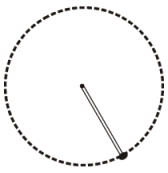Fig 32. The possible positions of a single pendulum are described by the points of a circle. We can describe the possible positions of a double pendulum by two circular coordinates. So in that case we can represent the mathematical space of of all these positions by a torus ie a doughnut shape, since any point on a torus is also described by two circular coordinates. In science, a mathematical space describing possible states of a system is called a phase space. It is an important concept in the mathematical modelling of phenomena in the real world. (Note: the complete phase space of a double pendulum would also include the momentum and thus be higher dimensional).Fig 33.
 Fig 34. The phase space of the movement of the double pendulum is described by a path on a torus (we can call this more accurately the positional space). This movement is in fact chaotic, as illustrated by this animated (in html) diagram by Eric Weisstein (World of Physics).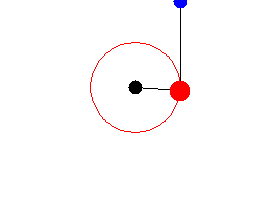Fig 35. An example of such a path is a trefoil knot on a torus as illustrated. It represents a movement of the double pendulum in which one part goes round 3 times while the other goes round twice and this path is called a (3,2)- torus knot.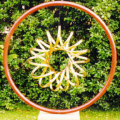Fig 36. I also show a another example of a path on a torus: a sculpture by John Robinson called "Rhythm of Life" (left) which he describes as representing DNA spinning within the universe. Within a circle, representing the universe, is a band of bronze which goes 15 times one way and 4 times the other - a (15,4)-torus knot.

Now we can turn the situation round. Instead of using mathematical space to study and represent motion, we can use motion, i.e. paths, to study mathematical space, and to see how spaces can differ. As an illustration of the method, I use knot spaces.

#### Paths and knot spaces

In Fig 37 we picture a pentoil knot and we also show a path around it. To study the space around the knot, we can fly around it. As we do so, it is convenient to carry a piece of string with us, to record how we have travelled. This string will represent a mathematical path in our space around the knot, and if the path returns back to itself, we call it a loop.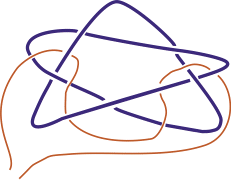Fig 37. Pentoil with path.Fig 38. Loop X around the knot X.
 In order to show precisely how we move around the knot, we put an arrow on the knot, and label each part of the diagram, and then give the same label to a loop around that part (Fig 38). Notice that if we make one loop, then we can make another, and so obtain a product of loops (Fig 39). Since the string is floppy, and the knot is rigid, it is sensible to regard two loops as the same if we can move one into the other. As in our video, we are interested in the classification of loops, where two loops are the same if one can be deformed into the other.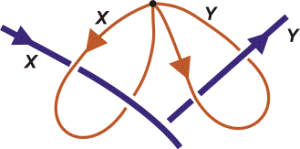Fig 39. Product of loops.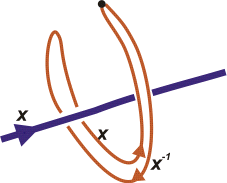Fig 40. Inverse of a loop Each loop around the knot may be reversed, and in the classification of loops, the reverse gives an inverse class for the product of classes of loops. These classes of loops with their products form a group. This group is called the Poincaré group of the knot space since it was first studied by him in connection with classifying motions of three bodies under the influence of gravity. It was one of the tools he developed for studying high dimensional spaces (up to dimension 17 in the case of the three body problem!) and in this way gave a foundation for what is now called algebraic topology. We can now study relations or rules in this group of the pentoil knot. We divide the diagram of the knot into overpasses - thus the pentoil has 5 overpasses (Fig 41). We label these overpasses and give them a direction. We use the same labels for a string (represented by the pink lines) passing round or under the overpass in the right direction. So in fig 42, we show how a string going under the overpass y can be moved to a string going under x, under z and back (ie. inverse) under x, which gives us: z =xyx-1.Fig 41. Pentoil with labels and arrows.Fig 42. Passing an overpass. Note here the lack of commutativity. If in this equation we could move x past y , then the x and x-1 would cancel and we would have z = y, suggesting the string would move pass the crossing, which experiment shows cannot happen. If we write rules of this type for each crossing in a diagram of a pentoil and then manipulate the equations algebraically, eliminating all letters except x and y , we end up with the strange equation or relation between x and y : xyxyxyx-1x-1y-1x-1y-1 = 1 . This relation is not an abstraction! If we tie our string on the knot according to this rule (Fig 43), and then tie the ends of the string together, we seem to have it well tied on. But some movements and wiggling shows that it comes off! This formula is a kind of invariant of the knot. More precisely, the letters x and y , and the relation, define an abstract group, the group of the knot, and if two knot spaces give rise to formulae defining different groups, then the knots are different. Thus this method gives a way of solving the difficult problem of distinguishing knots.Fig 43. Tying on string according to a rule.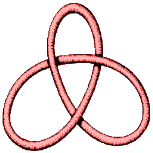Fig 44. Trefoil knot. Different versions of the pentoil have the same group, but a different knot may have a different group. For example, for the trefoil knot (fig 44) we get xyxy-1x-1y-1 = 1 while for most knots it is not possible to reduce the relations for the group to one involving only two unknowns.

This idea of constructing invariants of a space in the form of abstract groups, or other kinds of algebraic structures, is an important tool for studying geometric problems, and a part of the subject of topology (Topology : the study of the geometry of continuous deformations: - aka "rubber sheet" geometry) is to find such invariants, describe their properties, and calculate them for specific examples. There is an important mathematical principle involved at this point. The fact that the string comes off the knot proves that the relation holds. However, if the string has not come off after some amount of trying this does not prove that it will not come off. So to prove that the trefoil formula will not work for the pentoil, and vice versa, needs some real mathematical work. What is needed is the 1-dimensional Van Kampen Theorem referred to earlier! This illustrates that to prove that something cannot be done is often a difficult and sophisticated procedure. A further point is that there are many different ways of taking the string off the knot.

And so we move towards higher dimensions: the classification of these "proofs" that the relation holds is an example of a higher dimensional problem, requiring a new range of methods.Top : Table of Contents.Next page: To Higher Dimensions...Previous Page: Introduction, Symmetry, ...Home

View My Stats